## Table of ContentOpen Access

ARTICLE

# Prediction of Link Failure in MANET-IoT Using Fuzzy Linear Regression

R. Mahalakshmi1,*, V. Prasanna Srinivasan2, S. Aghalya3, D. Muthukumaran4

1 Department of Electrical and Electronics Engineering, Dhanalakshmi Srinivasan College of Engineering and Technology, Mamallapuram, Tamil Nadu, India
2 Department of Information Technology, R.M.D. Engineering College, Kavaraipettai, Chennai, Tamil Nadu, India
3 Department of Electronics and Communication Engineering, St. Joseph’s College of Engineering, Chennai, Tamil Nadu, India
4 Department of Electronics and Communication Engineering, Sri Chandrasekharendra Saraswathi Viswa Mahavidyalaya University, Kancheepuram, Tamil Nadu, India

* Corresponding Author: R. Mahalakshmi. Email:Intelligent Automation & Soft Computing 2023, 36(2), 1627-1637. https://doi.org/10.32604/iasc.2023.032709

## Keywords

1  Introduction

In the present world of technology, several physical things can be utilized to facilitate human work . That is why the IoT, a modern approach and a better solution that allows the association of physical objects with the digital world by exploiting networks and transmission technologies, is used. The IoT in smart surroundings interrelates with MANET, assembly it even more attractive to the users and reasonably successful. Interaction between MANETs with the IoT permits the creation of novel MANET-IoT systems.

The Fuzzy Linear Regression Method (FLRM) analysis is a dominant tool for examining and forecasting data by measuring a vague concept, which contains a degree of ambiguity and uncertainty . Applying FLRM to the experimental interpretation has allowed several phenomena in many fields of science such as chemistry, agriculture, environment, medicine, biology, psychology, and economics. These techniques necessitate an adequate number of accurate and reliable observations. A fuzzy regression is an input-output relative in the fuzzy numbers . Contrast the classic linear regression in that the parameters are unspecified to be random variables with possibility allocation functions in a fuzzy regression. In a fuzzy regression model, the data of input (X) and the output (Y) represents the fuzzy, relationship between the input and output data is specified by a fuzzy function . The benefits of this model is programming simplicity, and lesser deviation between the observed and evaluated values. PSO algorithm is a simple and fast optimizer that can solve real-world optimization issues . This algorithm improves the efficiency of searching and it minimizes the network overhead. The PSO algorithm contains four modules as parameters of PSO controlling, PSO hybridizing, meta-heuristic methods, coordination and multi-swarm methods . It minimizes the delay and raises the success rate. HC is a heuristic method that reach the optimal solution with faster convergence in a smaller computational time . HC method is used to improve the search capability and it efficiently discovers the GLs in the network.

Problem Statement:

A Least Square Quadratic Polynomial Regression based method (LSQPR) evaluates the link failure time by applying the signal strength . The forwarding and obtaining node’s distance is computed from the data packets received signal strength. The authors have generated an optimal error quadratic model between this node distance and data packet arrival time. The formulation of the quadratic model and the time of link failure are measured to discover the moment at which the greatest transmission range will be attained for regard as the pair of transmitter-receiver. However, this approach can’t detect the link failure accurately. Furthermore, it can’t provide a better QoS alternative path in the network.

The link reliability and performance optimization approach apply a cost function that linearly merges the ratio of node energy, reliability of link, and precise incorporation rate operations . This approach involves a reliable link routing policy and the contention window techniques adjustment. This method enhances the lifetime by minimizing the node energy utilization. But, this approach can’t perform well. As a result, this work prediction of link failure in MANET-IoT using fuzzy linear regression is proposed. The contributions of this approach are as follows:

•   The FLRM is used to forecast the link life competently in MANET by distance and time as the input parameters. The FLRM measures the long lifespan link based on the link failure prediction.

•   Initially, MANET forms the groups by the node received signal strength. Then, selects the GL based on the HC method. HC method is a optimal local search algorithm that selects the GL by node mobility, link energy, and link distance between nodes.

•   PSO is a random path solution that searches for optima through updating generations. The PSO algorithm selects the optimal DG nodes based on the node mobility and energy in the MANET.

•   The simulation results demonstrate that the FLRLF approach increases the GL lifespan and minimizes the link failure time in the MANET.

The structure of the remaining parts of the article is as follows: Section 2 describes related work. Section 3 describes the fuzzy linear regression method. Section 4 explains the FLRM to measure the link failure and provide an optimal route in the IoT MANET. Next, simulation results are specified in Section 5, and the article’s conclusions in Section 6.

2  Related Works

A fuzzy-based enhanced CH selection approach uses a Grey Wolf Optimizer (GWO) method to choose the Cluster Heads (CHs) . GWO is an intelligence algorithm through the action of grey wolves. It selects the CH’s based on every node energy utilization and presents the remaining energy. This approach balances energy utilization during the remaining nodes utilize single-hop transmission. However, this approach increases the CH routing overhead, and CH is easily dead. An improved energy-efficient clustering approach is used to extend the lifespan . The optimal amount of clusters is decided by the corresponding balanced clusters built by the fuzzy c-means to minimize and balance energy utilization. Then CHs are chosen in the best positions with CH replacement operation by incorporating a back-off timer method for CH selection.

A time synchronization approach is an efficient and simple method for time synchronization . Two compressive data gathering approach is used to balance the projection nodes. Even clustering method based on spatial position is introduced to disseminate the projection nodes consistently and equate the energy utilization. An even clustering by density, considering the position and node density, equating the network energy and then extending the network lifetime is discussed in . An enhanced clustering hierarchy approach is introduced to attain energy efficiency by applying the sleeping-waking method . This approach reduces data redundancy and enhances the network lifetime. An energy-efficient clustering technique’s objective is to enhance energy efficiency by minimizing and equating energy utilization . The lemma concerning the dual-CH method is used to minimize the overhead of energy. A non-cooperative game method aims to balance the energy utilization among the CHs. A fusion of Ant Colony Optimization (ACO) and Cuckoo Search (CS) techniques is introduced in energy-efficient routing . Here, ACO is applied for discovering leader nodes then the CS technique is used for data forwarder node selection during data transmission. However, this approach lacks energy efficiency during multi-hop.

An improved artificial bee colony method to select the CH by node density, node energy, and node position is discussed in . The fuzzy C-means clustering method is employed for forming the clustering. Then form the energy-efficient routing algorithm by ACO between the CH and the sink. This approach enhances the network throughput. A Link quality evaluation expresses the link quality, and this approach is unstable . An RSSI-based link quality measurement is used to enhance the quality . However, it creates additional computational complexity.

3  Fuzzy Linear Regression Method

A network contains a group of mobile nodes which transmit the data through a regular disseminate channel with a similar communication range . The major function of FLRM is to determine the best method with the slightest fault. FLRM can be categorized into two classes: the Possibility approach and the Least squares model.

The possibility approach attempts to reduce an entire fuzziness by diminishing the whole spreads of its fuzzy coefficients, plus the data of every sample inside a specific possible data interval . The parameter evaluation is a linear programming issue; here, the inputs are crisp, and the outcome is a number of fuzzy. Afterward, the optimization is solved so that the interpretation falls in the fuzzy sets. It attempts to decide the association between the value of the threshold and the input data while the data consist of a level of uncertainty. They utilized the threshold value to evaluate fitness degrees in an FLRM. The least-squares model reduces the sum of squared errors in the evaluated value by their specifications.

The FLRM illustrates the confidence of the output variable in the input variables.

Y=f(x,B)=B0,B1x1++Bnxn (1)

Here, Y represents the fuzzy output, X=[X1,X2Xn]T indicates the real input vector, and A=[B0,B1An] is the rest of the fuzzy numbers with the subsequent regular triangular membership functions ( ωBi(bi) ).

ωBi(bi)={1|bicbi|biS|bicbi|biS0otherwise (2)

Here, bic represents the centre value of Bi and biS indicates the multiply around the value of centre. The parameter of fuzzy B can be denoted as

B=(bcbs) (3)

Here, bc=(b1c,bnc)T and bs=(b1S,bnS)T . Next, the regression problem is defined as the specified set of crisp monitored values based on the many criteria of the integrity of the fit. The evaluated outcome Y can be received by applying the n expansion rule.

Y=f(x,A)=(fc(x),fs(x)) (4)

Here, the centre of Y represents the fc(x) and it described as

fc(x)=b0c+b1cx1++b1cxn (5)

In addition, the fc(x) is described as

fs(x)=b0s+b1s|x1|++b1sxn (6)

The membership function of Y is ωy(y) mentioned as

ωy(y)=1|ybcxTb0c|bs|x|T+b0s

|bcxT+b0cy|bs|x|T+b0c

Here,

ωy(y)=0

otherwise,

|x|T=(|x1|,|xn|)T (7)

In order to obtain the fuzzy regression with minimized fuzziness, the aim function is to reduce the total spread of the fuzzy number.

4  FLRM to Measure the Link Failure and Provide an Optimal Route in the IoT MANET

The FLRM is used to forecast the link life competently in MANET by distance and time as the input parameters. The node’s position and time may be accessible honestly through correspondence with a satellite if nodes are prepared with a less power Global Positioning System (GPS). From position information, the distance among neighboring nodes can be computed. Since, in MANET, two node-link life correlates to the speed and movement direction between nodes, this is difficult to utilize numerical linear regression. The associations between distance and time variables in this situation are evaluated by utilizing the FLRM method.

In this approach, the data packets are forwarded from sender S to receiver R using the following pattern.

{S=b0,b1,bk1,bk=R} (8)

Here, b0,b1 are nodes and the route link is (bi1,bi),i=1,2,k. The lifespan of (bi1,bi) is unsure, and Xi denotes the lifespan. Let X1,X2,Xk are independent and identically disseminated random variables, each with mean DL(t) . The lengthier route from sender to receiver obviously happens in a MANET. A link breakage of a link on the route will cause origin failure.

The lifespan of a path P, which contains k wireless links, is unsure and utilizes an arbitrary variable Y to denote the lifespan. The value Y is described as

Y=min(x1x2xk) (9)

The dependability of the link DL(t) computation is given below

DL(t)=p{min(x1,x2xk)>t} (10)

The rate for the failure of the link represents the Fa(t) computation below.

Fa(t)=i=1kfi(t) (11)

The dependability of the link computation is given below.

DL(t)=exp{0ti=1kfi(u)du} (12)

Then, the lifespan threshold LSTH computation is given below.

LSTH=DL(t)kK (13)

where K denotes the hop count. To minimize link failure, the lengthier life route in the MANET is identified. Fig. 1 demonstrates the structure of the proposed scheme.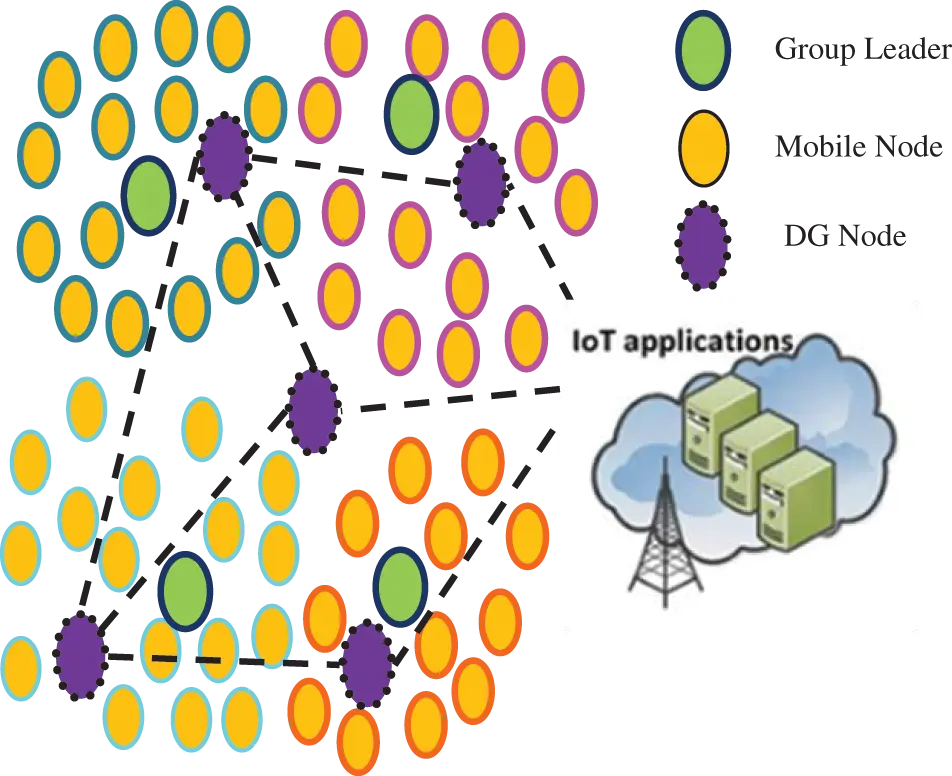Figure 1: Structure of the proposed scheme

The MANET contains a number of mobile nodes, these nodes move randomly, and nodes have equal communication ranges. In MANET, The grouping method is executed to minimize energy utilization. A node which is associates together is known as a group. GL is an essential cooperative function for a network. In a grouped MANET, a node is selected as a GL that collects its group data and then it transmits to the sink through DG nodes. This approach using DG nodes for collecting data from the GL, as a result, minimized the GL burden in the MANET. Initially, the mobile nodes formed the group by the node received signal strength. Then, the GL is selected by the HC method.

HC is an optimization method that goes to the local search. It provides a large set of inputs and a the best heuristic operation, it tries to discover an adequately good solution to the problem. It explores the best resolution in the neighborhood by measuring the present state. Otherwise, continue updating the current state, if possible. The fundamental concept of HC is forever a condition that is best than the present one. Hence, it enhances the quality. HC method chooses the GL by the node mobility, link energy, and link distance between nodes. These parameters’ value is greater than the threshold value that node is selected as a GL. Fig. 2 shows the selection of GL by HC algorithm.Figure 2: Hill climbing method

The procedure of the HC method is as follows:

1.    Collect the Sender Neighbor nodes as a crowd nest.

2.    Select a mobile node through levy flight and estimate its fitness F.

3.    Equate Fitness with the threshold value.

4.    If Fit (Link Lifespan) > Threshold value, the node is taken a chance as a GL node;

5.    If Fit (Link Energy) > Threshold value, the node is taken a chance as a GL node;

6.    If Fit (Link Distance) > Threshold value, the node is taken a chance as a GL node;

7.    if new node > present node

8.    Select a new node as a GL node

After selecting the GL, the GL collects the information from the mobile nodes and forward it to the sink node through the DG node. Next, the GL node forwards the collected data to the DG, and transmits the data to the sink via the intermediate DG. This DG node minimizes the network’s GL energy utilization and GL burden. The PSO algorithm selects the optimal DG nodes based on the node mobility and energy in the MANET.

PSO is a random path solution that searches for optima through updating generations. In each iteration, each node is updated by following two best values such as Lbest and gbest. The first one is the best node fitness value among neighbors is called Local best (Lbest). Another best value tracked by the particle swarm optimizer is the best value received so far through any node in the path. This best value is a global best (gbest). When a particle takes part of the population as its topological neighbors.

After discovering the two best values, the node adapts its node fitness value with the following formulas.

V[]=V[]+h1arbit()(Lbest[]current[])+h2arbit()(gbest[]current[]) (14)

current[]=current[]+V[] (15)

where,

V[] → node fitness value,

current[] → recent node

arbit() → arbit number between (0, 1)

h1,h2 → learning factors. Usually h1 = Link Lifespan and h2 = Energy

The lowest mobility and highest energy node is selected as a DG node in the MANET.

5  Results and Discussion

In this work, the network simulator-2.35 tool is used to measure the network function of FLRLF and LSQPR approaches. A total of 100 mobile nodes are used and these nodes’s transmission range is set to 250 metre. These approaches network simulation time is 200 s. Random waypoint mobility to create the FLRLF scenario mobile nodes movement velocity is 4 to 20 m/s. Fig. 3 explains the packet forward ratio of LSQPR and FLRLF based on node velocity.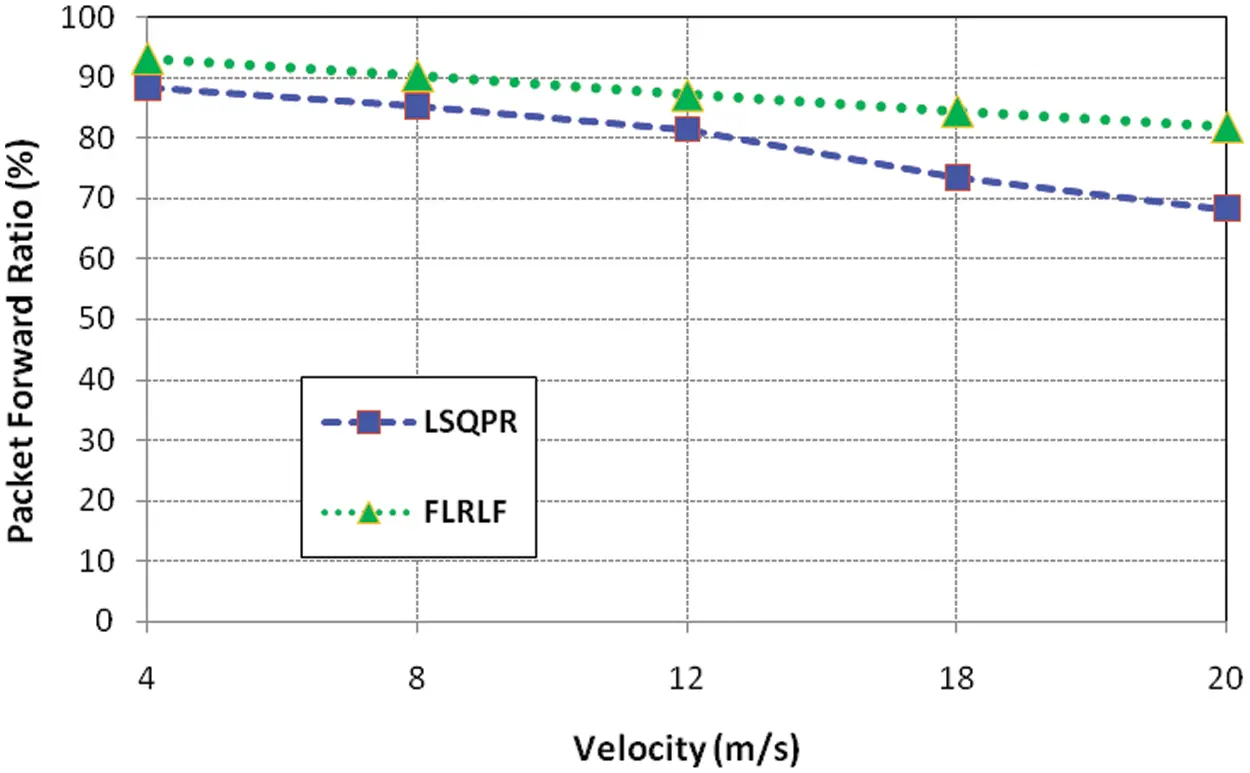Figure 3: Packet forward ratio of LSQPR and FLRLF based on node velocity

Packet forward ratio denotes the amount of packets efficiently forward to the sink node. The ratio of packets forward is higher in the FLRLF approach equated to the LSQPR approach, as shown in Fig. 3, since the FLRM minimizes the link failure reduction in the MAENT. Fig. 4 demonstrates the packet drop ratio of LSQPR and FLRLF based on node velocity. Packet drop ratio denotes the amount of packets dropped during data transmission from sender to sink node.Figure 4: Packet drop ratio of LSQPR and FLRLF based on node velocity

It can be seen from Fig. 4 that the FLRLF approach’s packet drop ratio is lower than the LSQPR approach. Due to the FLRLF method using the PSO and HC, optimal search algorithms are enhancing the routing efficiency. But, LSQPR can’t detect the link failure accurately in the MANET. Fig. 5 represents the delay of LSQPR and FLRLF based on node velocity.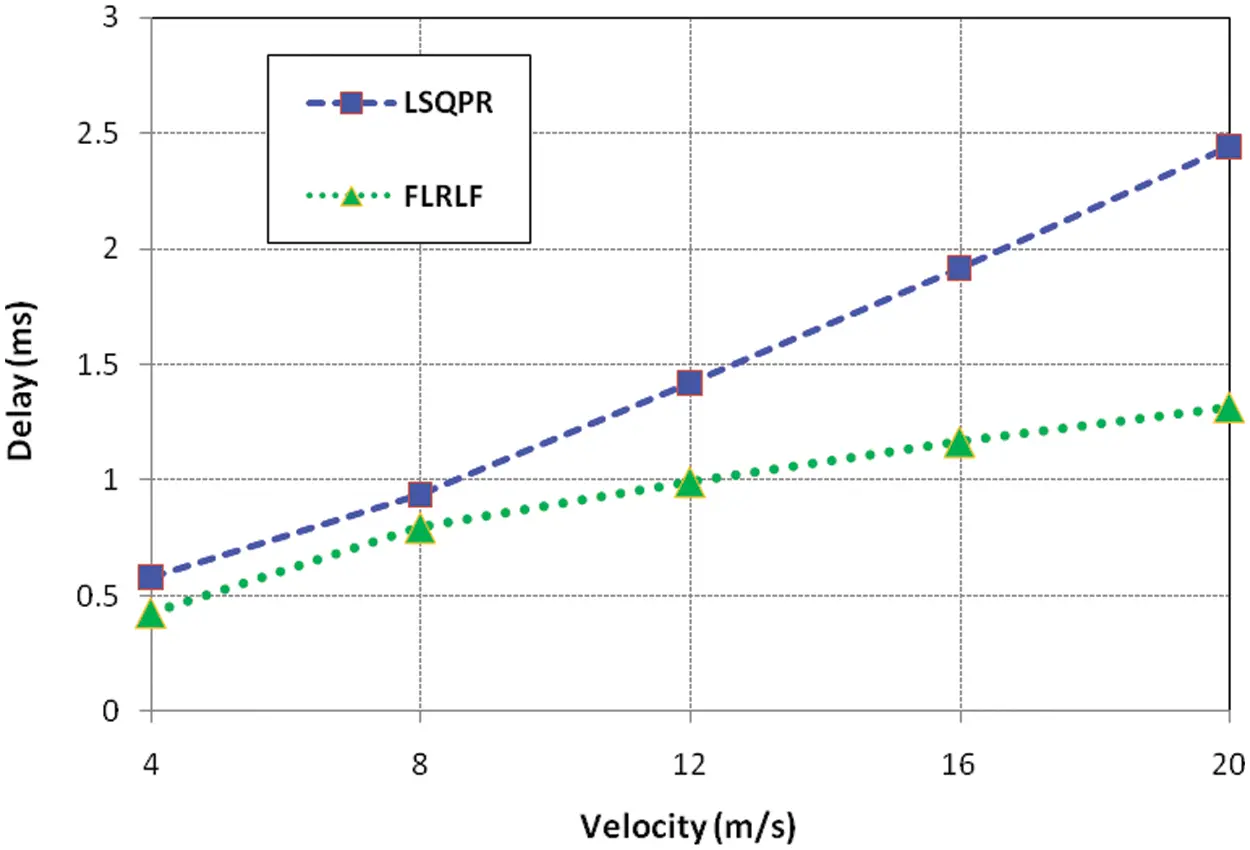Figure 5: Delay of LSQPR and FLRLF based on node velocity

The delay represents the difference in a packet delivered time and receives time for every node. Fig. 5 illustrates that the FLRLF has a lesser delay compared to the LSQPR approach. Since the DG node gathers the information from the GL; as a result, FLRLF minimized the network delay. Fig. 6 illustrates the GL lifespan of LSQPR and FLRLF approaches based on node velocity.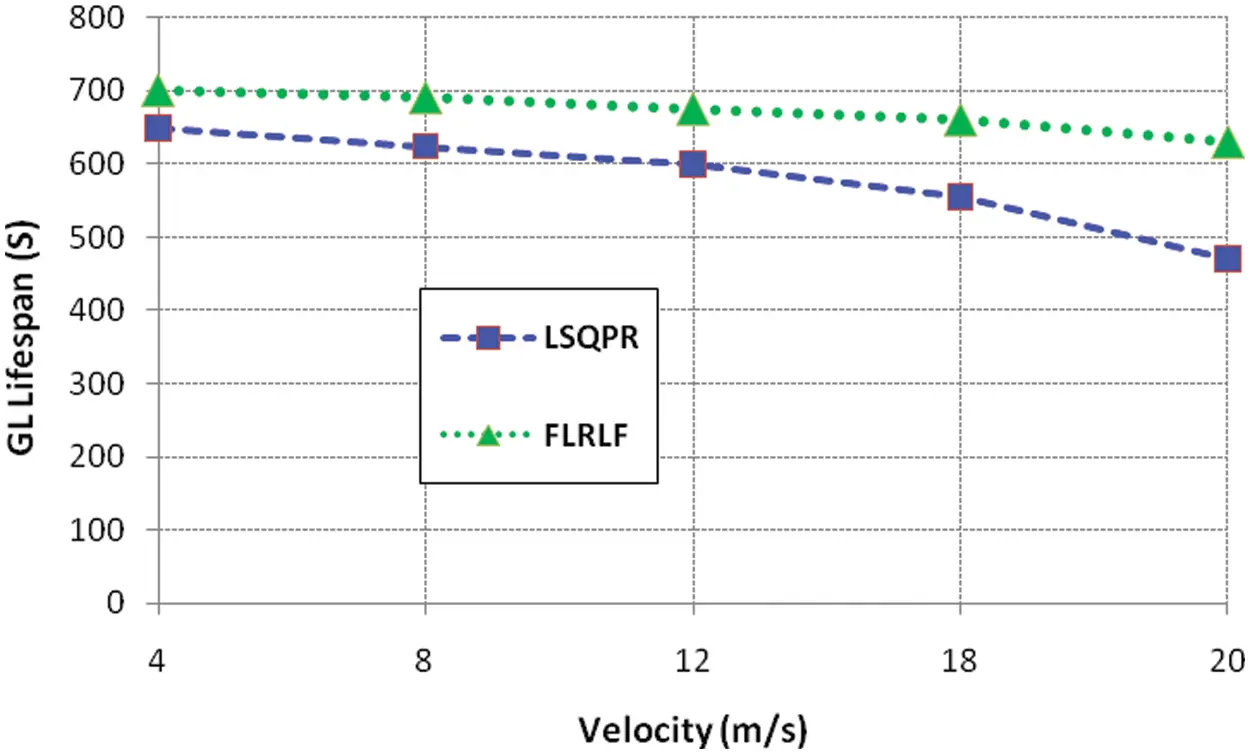Figure 6: CH lifespan of LSQPR and FLRLF based on node velocity

As node velocity rises, the GL lifespan of LSQPR and FLRLF will drop. This represents that the node velocity severely affects the network lifespan. However, the FLRLF approach GL lifespan is nearly 630 s. But, LSQPR lifespan is below 500 s. Fig. 7 explains the link failure time of LSQPR and FLRLF based on node velocity.Figure 7: Link failure time of LSQPR and FLRLF based on node velocity

From Fig. 7, the node velocity raises the link failure also raised. The LSQPR increases the velocity and the LSQPR link failure time is also highly raised. However, the FLRLF approach little raises the link failure time. Since the FLRLF calculates the link lifespan by FLRM. Fig. 8 demonstrates the remaining energy of LSQPR and FLRLF based on node velocity.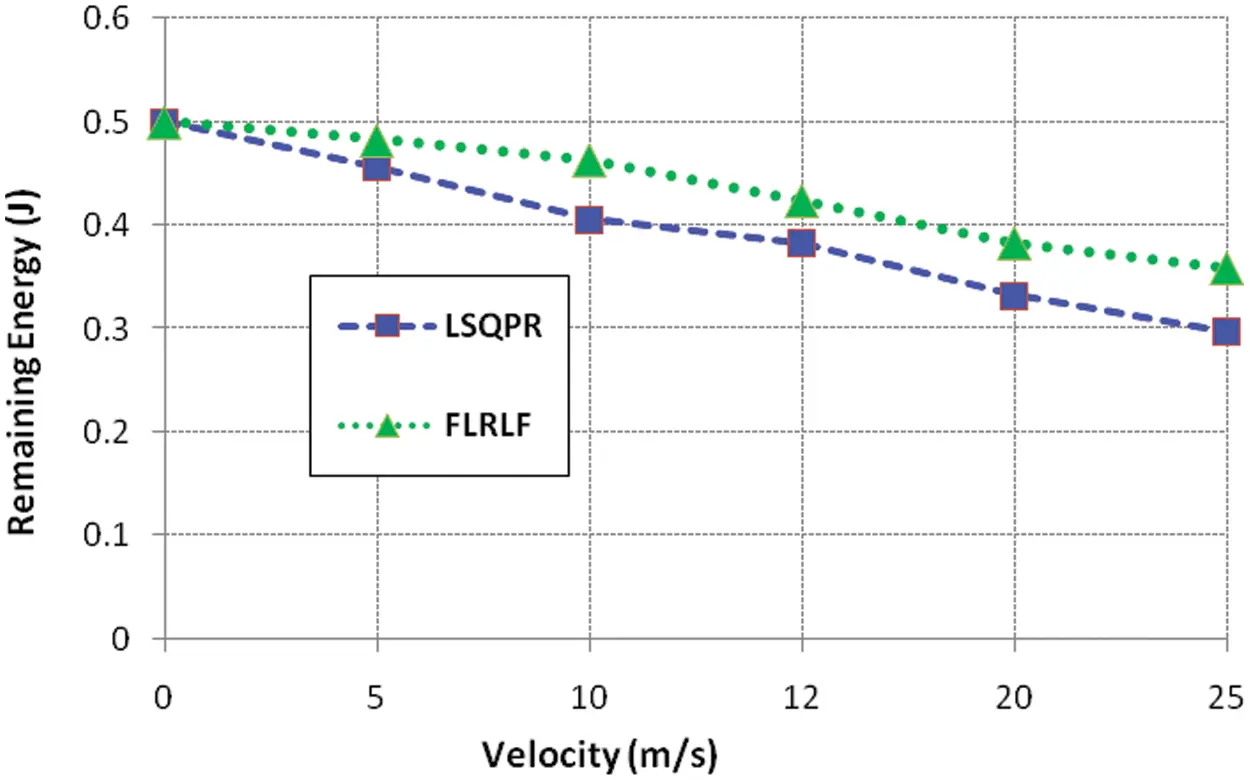Figure 8: Remaining energy of LSQPR and FLRLF based on node velocity

During data transmission LSQPR approach highly utilized energy. But, the FLRLF approach preserves the energy in the MANET. The DG node avoids the GL dead issues and minimizes the network delay.

6  Conclusions

Funding Statement: The authors received no specific funding for this study.

Conflicts of Interest: The authors declare that they have no conflicts of interest to report regarding the present study.

## References

1. R. Bruzgiene, L. Narbutaite and T. Adomkus, “MANET network in internet of things system,” Ad Hoc Networks, vol. 66, pp. 89–114, 2017.
2. K. B. Song, Y. S. Baek, D. H. Hong and G. Jang, “Short-term load forecasting for the holidays using fuzzy linear regression method,” IEEE Transactions on Power Systems, vol. 20, no. 1, pp. 96–101, 2005.
3. E. Hosseinzadeh and H. Hassanpour, “Estimating the parameters of fuzzy linear regression model with crisp inputs and Gaussian fuzzy outputs: A goal programming approach,” Soft Computing, vol. 25, no. 4, pp. 2719–2728, 2021.
4. M. M. Nasrabadi and E. Nasrabadi, “A mathematical-programming approach to fuzzy linear regression analysis,” Applied Mathematics and Computation, vol. 155, no. 3, pp. 873–881, 200
5. D. Kumar and M. Pandey, “An optimal and secure resource searching algorithm for unstructured mobile peer-to-peer network using particle swarm optimization,” Applied Intelligence, vol. 2022, pp. 1–18, 2022.
6. T. M. Shami, A. A. El-Saleh, M. Alswaitti, Q. Al-Tashi, M. A. Summakieh et al., “Particle swarm optimization: A comprehensive survey,” IEEE Access, vol. 10, pp. 10031–10061, 2022.
7. N. S. Kavitha and G. Singaravel, “Balanced wireless sensor networks with energy efficient bees swarm–harmony search–hill climbing mechanism,” International Journal of Communication Systems, vol. 34, no. 8, pp. e4781, 2021.
8. S. Patel and H. Pathak, “A mathematical framework for link failure time estimation in MANETs,” Engineering Science and Technology, an International Journal, vol. 25, pp. 1–13, 2022.
9. K. G. Mkongwa, Q. Liu and C. Zhang, “Link reliability and performance optimization in wireless body area networks,” IEEE Access, vol. 7, pp. 155392–155404, 201
10. K. Jaiswal and V. Anand, “A grey-wolf based optimized clustering approach to improve QoS in wireless sensor networks for IoT applications,” Peer-to-Peer Networking and Applications, vol. 14, no. 4, pp. 1943–1962, 2021.
11. H. Safa, O. Mirza and H. Artail, “A dynamic energy efficient clustering algorithm for MANETs,” in IEEE Int. Conf. on Wireless and Mobile Computing, Networking and Communications, Avignon, France, pp. 51–56, 2008.
12. R. H. Hwang, C. Y. Wang, C. J. Wu and G. N. Chen, “A novel efficient power-saving MAC protocol for multihop MANETs,” International Journal of Communication Systems, vol. 26, no. 1, pp. 34–55, 2013.
13. L. Liu, C. Chen, T. Qiu, M. Zhang, S. Li et al., “A data dissemination scheme based on clustering and probabilistic broadcasting in VANETs,” Vehicular Communications, vol. 13, pp. 78–88, 2018.
14. S. Shruthi, “Proactive routing protocols for a MANET—A review,” in Int. Conf. on I-SMAC (IoT in Social, Mobile, Analytics and Cloud), Palladam, India, pp. 821–827, 2017.
15. K. Jain, A. Kumar and C. K. Jha, “Probabilistic-based energy-efficient single-hop clustering technique for sensor networks,” in Int. Conf. on Communication and Intelligent Systems, Jaipur, India, pp. 353–365, 2019.
16. G. Devika, D. Ramesh and A. G. Karegowda, “An energy-efficient routing and compression based data collection applying bio-inspired ant-cuckoo technique for wireless sensor network,” in Int. Conf. on Computational Systems and Information Technology for Sustainable Solution, Bengaluru, India, vol. 4, pp. 1–8, 2019.
17. S. Ghosh and S. K. Dubey, “Comparative analysis of k-means and fuzzy c-means algorithms,” International Journal of Advanced Computer Science and Applications, vol. 4, no. 4, pp. 35–39, 2013.
18. I. A. Basheer and M. Hajmeer, “Artificial neural networks: Fundamentals, computing, design, and application,” Journal of Microbiological Methods, vol. 43, no. 1, pp. 3–31, 2000.
19. P. Mishra, C. Gandhi and B. Singh, “Link quality and energy aware geographical routing in MANETs using fuzzy logic,” Journal of Telecommunications and Information Technology, vol. 2016, no. 3, pp. 5–17, 2016.
20. F. Entezami, M. Tunicliffe and C. Politis, “Find the weakest link: Statistical analysis on wireless sensor network link-quality metrics,” IEEE Vehicular Technology Magazine, vol. 9, no. 3, pp. 28–38, 2014.
21. C. X. Zhao and G. X. Wang, “Routing protocol based on fuzzy regression for MANET,” in Int. Conf. on Machine Learning and Cybernetics, Shanghai, China, vol. 3, pp. 1811–1815, 2004.

R. Mahalakshmi, V. Prasanna Srinivasan, S. Aghalya and D. Muthukumaran, "Prediction of link failure in manet-iot using fuzzy linear regression," Intelligent Automation & Soft Computing, vol. 36, no.2, pp. 1627–1637, 2023.This work is licensed under a Creative Commons Attribution 4.0 International License , which permits unrestricted use, distribution, and reproduction in any medium, provided the original work is properly cited.

View

Like

## Related articles

• RamanaM. Pidaparti
• Jin Wang, Chunwei Ju, Yu Gao,...
• Guo Zhao, Jiang Guo, Hao Qiang
• Balamati Choudhury, Pavani Vijay...
• Xiaoyi Liang, Zhen Yang, Xingsheng...